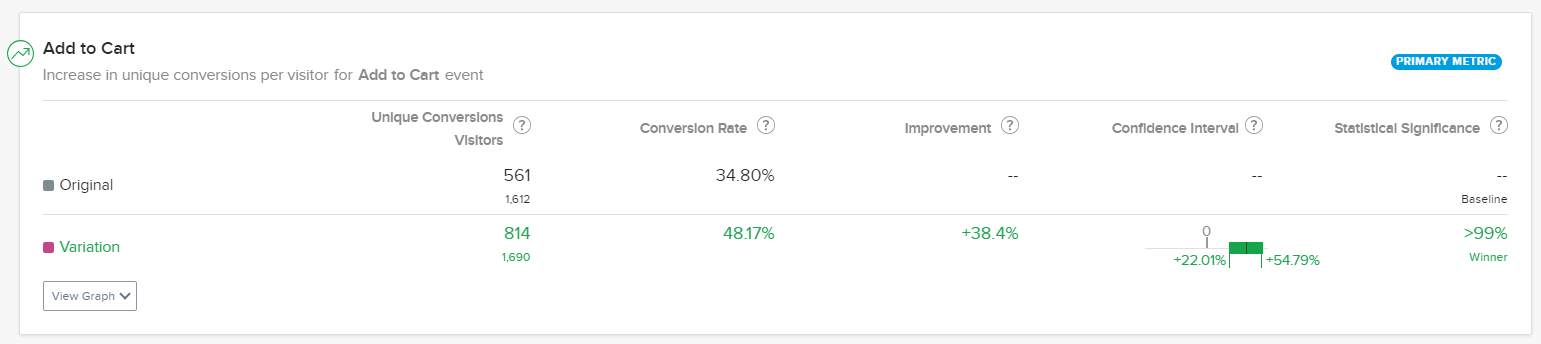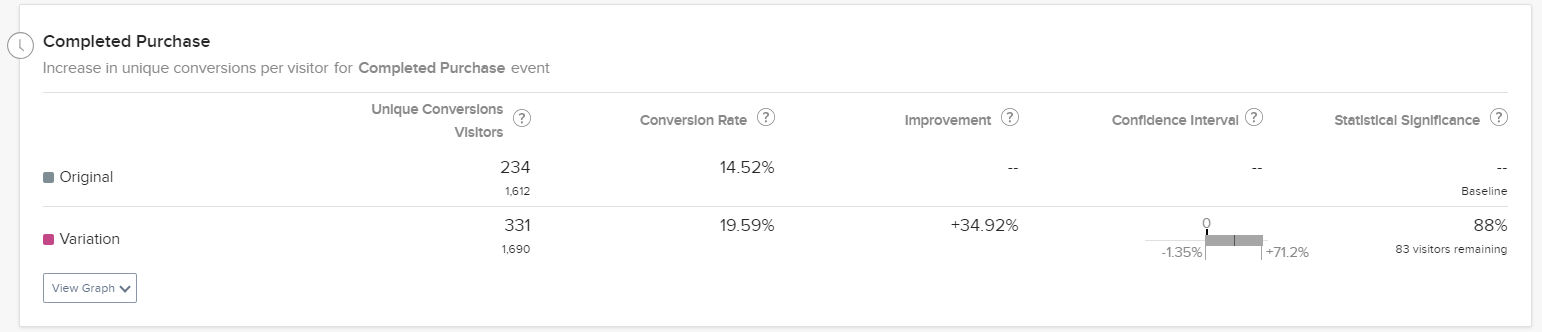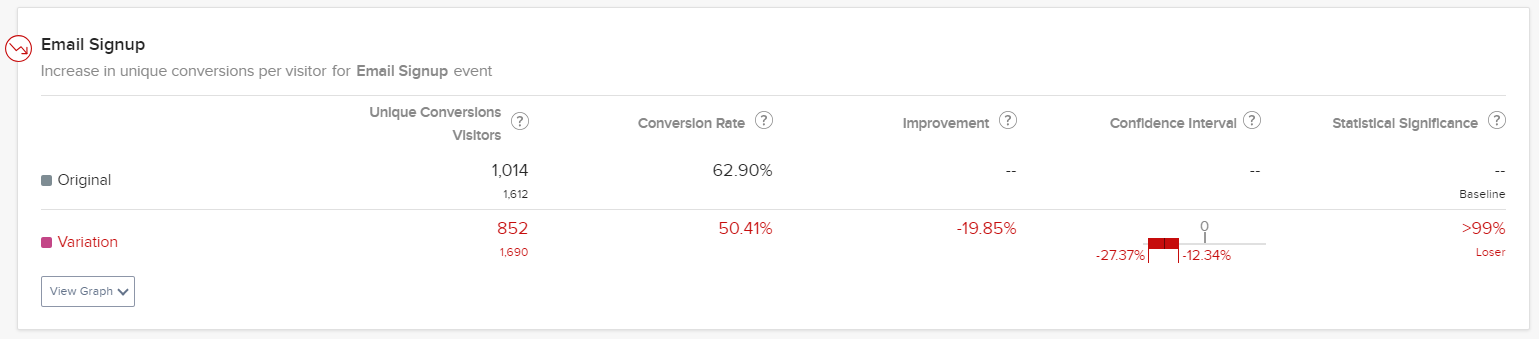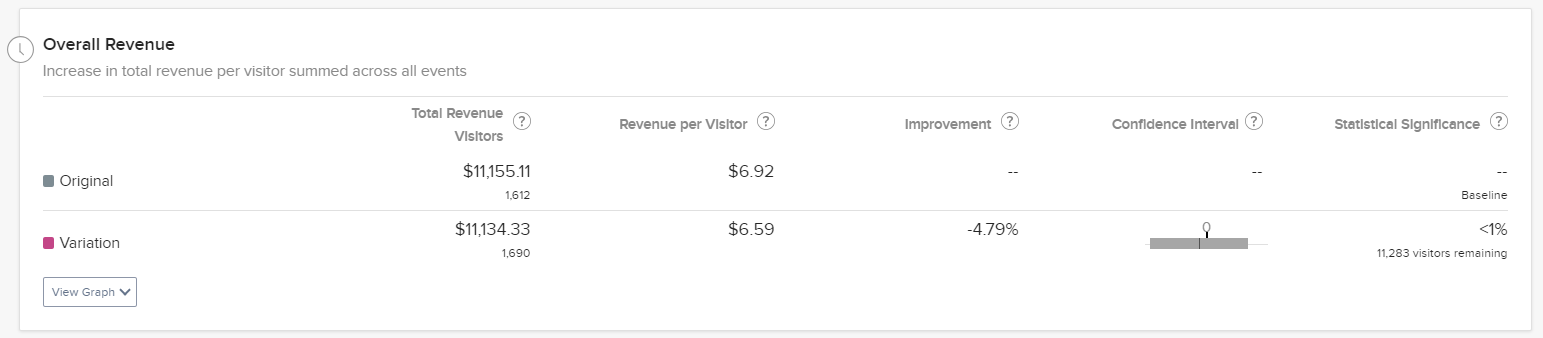# Confidence intervals and improvement intervals

• Updated
• Optimizely Web Experimentation
• Optimizely Performance Edge
• Optimizely Feature Experimentation
• Optimizely Full Stack (Legacy)

Statistical significance measures how unusual your experiment results would be if there were actually no difference in performance between your variation and baseline and the discrepancy in lift was due to random chance alone. Statistical significance gives you an idea of how strongly the data contradicts the baseline hypothesis of there actually being no difference between the test variation and the control.

## Confidence intervals

Confidence interval refers to an estimated range of values that are likely, but not guaranteed, to include the unknown but exact value summarizing your audience if you replicated an experiment numerous times. Confidence Intervals consider both the size of the sample exposed to an experiment and the amount of variability, that is dispersion or noise, in that experiment sample.

Confidence intervals contain:

• A point estimate (uplift or improvement) – A single value derived from your statistical model of choice.
• A margin of error – Around that point estimate that indicates the amount of uncertainty that surrounds the sample estimate from the population.

It is a best practice to report confidence intervals to supplement your statistical significance results, as they can offer information about the observed effect size of your experiment.

Optimizely Experimentation's confidence interval is adaptive. The results page shows the running intersection of all previous confidence intervals by tracking the smallest upper limit and the largest lower confidence interval limit values during the experiment runtime. The largest lower limit means the less negative value or the value closest to zero. The technique ensures optimal tradeoff of estimating the bias and the variance so you can feel confident about the result.

Optimizely Experimentation sets your confidence interval to the same level that you set your statistical significance threshold for the project. The statistical significance setting for your project is 90% by default.

## When Optimizely Experimentation declares significance

A variation is declared significant as soon as the confidence interval stops crossing zero. Intervals crossing zero mean there is not enough evidence to say if there is a clear impact or not.

Once a variation reaches statistical significance, the confidence interval always lies entirely above or below 0.

### Confidence interval entirely above zero percentIn the preceding screenshot, enough evidence has accumulated so far that it is highly unlikely that the improvement we observe here is due to random chance alone. But, the improvement Optimizely Experimentation measured (+38.4%) may be different from the exact improvement you see going forward. The confidence interval indicates that this test variation will have a positive impact in the long run. For this experiment iteration, the error bounds were between 22.01% and 54.79% improvement.

The statistical significance setting for this example is 90%, which can tell you whether the results are consistent with being due to chance. As Optimizely Experimentation collects more data, the confidence interval may narrow.

### Confidence interval includes zero percent

Suppose you must stop a test early or have a low sample size. In that case, the confidence interval will give you a rough idea of whether implementing that variation will have a positive or negative impact.

For this reason, when you see low statistical significance on specific goals, the confidence interval can serve as another data point to help you make decisions. When you have an inconclusive goal, the interval will look like this:This variation's conversion rates is between -1.35% and 71.20%. You can interpret the confidence interval as a worst-case, middle-ground, and best-case scenario. For example, we are 90% confident that the difference between the variation and baseline conversion rates is:

• Worst-case – -1.35%
• Middle-ground – 34.93%.
• Best-case – 71.20%

### Confidence interval entirely below zero percentIn this example, enough evidence has accumulated so far that it is highly unlikely that the negative improvement we observe here is due to random chance alone. The negative improvement Optimizely Experimentation measured (-19.85%) is likely to be what you see going forward. The confidence interval indicates that this test variation will have a negative impact in the long run. For this experiment iteration, the error bounds were between -27.37% and -12.34% improvement.

In this experiment, the observed difference between the original (62.90%) and variation (50.41%) was -12.49%, just within the confidence interval. If we rerun this experiment, the baseline and variation conversion rate difference will probably be in the same range.

## How statistical significance and confidence intervals are connected

Optimizely Experimentation shows you the statistical likelihood that the improvement is due to changes you made on the page, not chance. Until Stats Engine has enough data to declare statistical significance, the Results page will state that more visitors are needed and show you an estimated wait time based on the current conversion rate.

Lower significance levels may increase the likelihood of error but can also help you test more hypotheses and iterate faster. Higher significance levels decrease the error probability but require a larger sample.

Choosing the right significance level should balance the types of tests you are running, the confidence you want to have in the tests and the amount of traffic you receive.

Significance and confidence intervals are connected, but not the same. Your experiment reaches significance precisely when your confidence interval on improvement moves away from zero.

## Improvement intervals

Depending on what type of experiment or optimization you are running, Optimizely Experimentation will display a different improvement interval on the Results page.

• For A/B experiments – Optimizely Experimentation displays the relative improvement in conversion rate for the variation over the baseline as a percentage for A/B experiments. This is true for all A/B experiment metrics, regardless of whether they are binary or numeric conversions
• In Optimizely Experimentation, a relative improvement interval of 1% to 10% means that the variation sees between 1% and 10% improvement over the baseline. For example, if the baseline conversion rate is 25%, you can expect the variation conversion rate to fall between 25.25% and 27.5%.
• For Multi-armed Bandit (MAB) optimizations – Optimizely Experimentation displays absolute improvement.
• For A/B experiments or MAB optimizations with Stats Accelerator enabled – Optimizely Experimentation displays the absolute improvement.
MAB optimizations do not generate statistical significance.

## Estimated wait time and <1% significance

As your experiment or campaign runs, Optimizely Experimentation estimates how long it will take for a test to reach conclusiveness. This estimate is based on the current observed baseline and variation conversion rates. If those rates change, the estimate will adjust automatically.You may see a significance of less than 1%, with a certain number of visitors remaining. In statistical terms, this experiment is underpowered. Optimizely Experimentation needs to gather more evidence to determine whether the change you see is a true difference in visitor behaviors or chance.

Look at the variation in the preceding example. Approximately 11,283 additional visitors must be bucketed into that variation before deciding the conversion rates between the variation and the original. Remember that the estimate (11,283 visitors) assumes that the observed conversion rate does not fluctuate. If more visitors see the variation but conversions decrease, your experiment will probably take more time, which means the visitors remaining estimate will increase. Optimizely Experimentation will need fewer visitors if conversions increase to ensure the behavior change is real.

To learn more about the importance of sample size, see our article on how long to run an experiment.

<% if (previousArticle || nextArticle) { %>
<% if (previousArticle) { %>
<% if (previousTitle) { %>

#### <%= previousTitle %>

<% } %> <%= previousArticle.title %>
<% } %> <% if (nextArticle) { %>
<% if (nextTitle) { %>

#### <%= nextTitle %>

<% } %> <%= nextArticle.title %>
<% } %>
<% } %>
<% if (allItems.length > 1) { %>